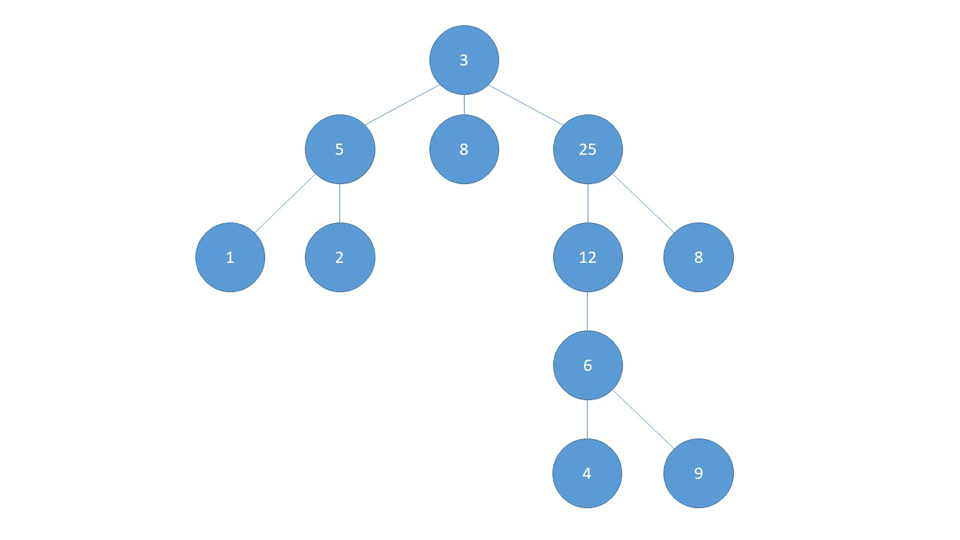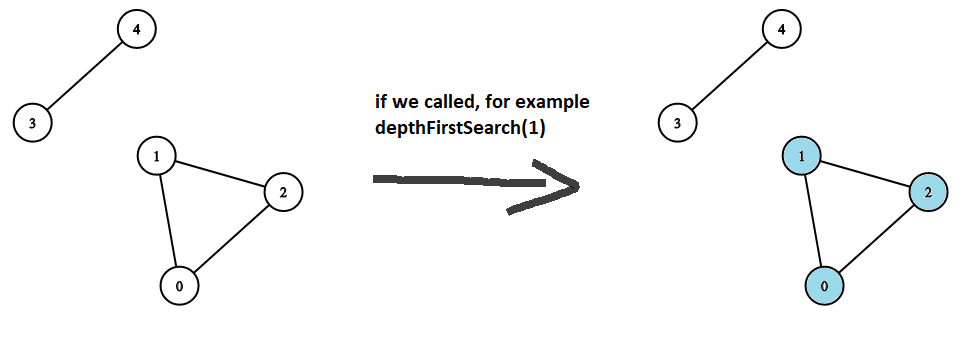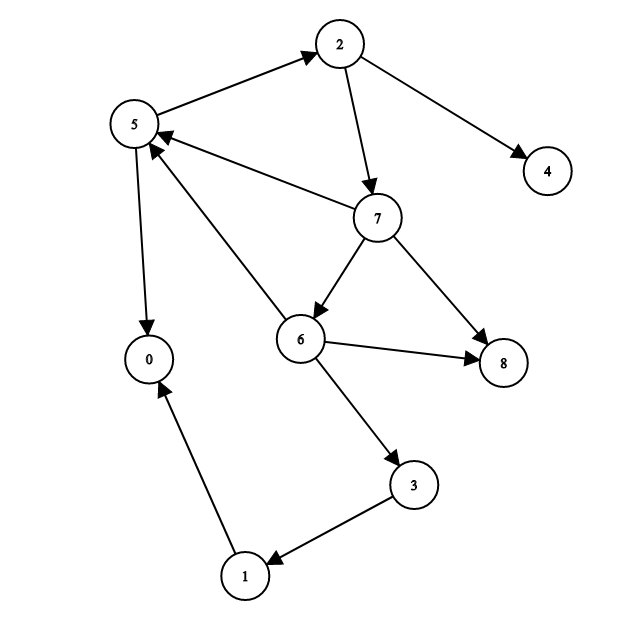Graphs in Java: Depth-First Search (DFS) - Stack Abuse

# Graphs in Java: Depth-First Search (DFS)### Introduction

Graphs are a convenient way to store certain types of data. The concept was ported from mathematics and appropriated for the needs of computer science.

Due to the fact that many things can be represented as graphs, graph traversal has become a common task, especially used in data science and machine learning.

### Depth-First Search

Depth-First Search (DFS) searches as far as possible along a branch and then backtracks to search as far as possible in the next branch. This means that in the proceeding Graph, it starts off with the first neighbor, and continues down the line as far as possible:Once it reaches the final node in that branch (1), it backtracks to the first node where it was faced with a possibility to change course (5) and visits that whole branch, which in our case is node (2).

Then it backtracks again to the node (5) and since it's already visited nodes (1) and (2), it backtracks to (3) and re-routes to the next branch (8).

### Implementation

Since we know how to represent graphs in code through adjacency lists and matrices, let's make a graph and traverse it using DFS. The graphs we'll be working with are simple enough that it doesn't matter which implementation we opt for.

Though, for actual projects, in most cases, adjacency lists will be a better choice, so we're going to represent the graph as an adjacency list.

We want to visit all our nodes once, as seen in the animation above they turn red once visited, so we don't visit them anymore. To do this in code, we'll introduce a visited flag:

public class Node {
int n;
String name;
boolean visited; // New attribute

Node(int n, String name) {
this.n = n;
this.name = name;
visited = false;
}

// Two new methods we'll need in our traversal algorithms
void visit() {
visited = true;
}

void unvisit() {
visited = false;
}
}


Now, let's define a Graph:

public class Graph {

// Each node maps to a list of all his neighbors
private boolean directed;

public Graph(boolean directed) {
this.directed = directed;
}

// ...
}


Now, let's add the method addEdge(). We'll use two methods, a helper method and the actual method.

In the helper method, we'll also make a check for possible duplicate edges. Before adding an edge between A and B, we'll first remove it and only then add it. If the edge already existed then this prevents us from adding a duplicate edge. If there was not already an edge there then we still only have one edge between the two nodes.

If the edge didn't exist, removing a non-existing edge will result in a NullPointerException so we're introducing a temporary copy of the list:

public void addEdgeHelper(Node a, Node b) {

if (tmp != null) {
tmp.remove(b);
}
}

public void addEdge(Node source, Node destination) {

// We make sure that every used node shows up in our .keySet()

// If a graph is undirected, we want to add an edge from destination to source as well
if (!directed) {
}
}


Finally, we'll have the printEdges(), hasEdge() and resetNodesVisited() helper methods, which are pretty straightforward:

public void printEdges() {
for (Node node : adjacencyMap.keySet()) {
System.out.print("The " + node.name + " has an edge towards: ");
for (Node neighbor : adjacencyMap.get(node)) {
System.out.print(neighbor.name + " ");
}
System.out.println();
}
}

public boolean hasEdge(Node source, Node destination) {
}

public void resetNodesVisited(){
node.unvisit();
}
}


We will also add the depthFirstSearch(Node node) method to our Graph class that does the following:

• If the node.visited == true, simply return
• If it hasn't been already visited, do the following:
• Find the first unvisited neighbor newNode of node and call depthFirstSearch(newNode)
• Repeat the process for all unvisited neighbors

Let's illustrate this with an example:

Node A is connected with node D
Node B is connected with nodes D, C
Node C is connected with nodes A, B
Node D is connected with nodes B

1. All the nodes are unvisited at the beginning (node.visited == false)
2. Call .depthFirstSeach() with an arbitrary node as the starting node, let's say depthFirstSearch(B)
3. mark B as visited
4. Does B have any unvisited neighbors? Yes -> the first unvisited node is D, so call depthFirstSearch(D)
5. mark D as visited
6. Does D have any unvisited neighbors? No -> (B has already been visited) return
7. Does B have any unvisited neighbors? Yes -> the first unvisited node is C, so call depthFirstSearch(C)
8. mark C as visited
9. Does C have any unvisited neighbors? Yes -> the first unvisited node is A, so call depthFirstSearch(A)
1. mark A as visited
2. Does A have any unvisited neighbors? No. -> return
10. Does C have any unvisited neighbors? No -> return
11. Does B have any unvisited neighbors? No -> return

Calling DFS on our graph would give us the traversal B,D,C,A (the order of visitation). When the algorithm is written out like this, it's easy to translate it to code:

## Free eBook: Git Essentials

Check out our hands-on, practical guide to learning Git, with best-practices, industry-accepted standards, and included cheat sheet. Stop Googling Git commands and actually learn it!

public void depthFirstSearch(Node node) {
node.visit();
System.out.print(node.name + " ");

if (allNeighbors == null)
return;

for (Node neighbor : allNeighbors) {
if (!neighbor.isVisited())
depthFirstSearch(neighbor);
}
}


Again, here's how it looks like when translated into an animation:DFS is sometimes called an "aggressive" graph traversal because it goes as far as it possibly can through one "branch". As we can see in the gif above, when DFS encounters node 25, it forces the 25 - 12 - 6 - 4 branch until it can't go any further. Only then does the algorithm go back to check for other unvisited neighbors of the previous nodes, starting with the ones more recently visited.

Note: We might have an unconnected graph. An unconnected graph is a graph that doesn't have a path between any two nodes.In this example, nodes 0, 1, and 2 would be visited and the output would show these nodes, and completely ignore nodes 3 and 4.

A similar thing would happen if we had called depthFirstSearch(4), only this time 4 and 3 would be visited while 0, 1, and 2 wouldn't. The solution to this problem is to keep calling DFS as long as there are any unvisited nodes.

This can be done in several ways, but we can make another slight modification to our Graph class to handle this problem. We'll add a new depthFirstSearchModified(Node node) method:

public void depthFirstSearchModified(Node node) {
depthFirstSearch(node);

for (Node n : adjacencyMap.keySet()) {
if (!n.isVisited()) {
depthFirstSearch(n);
}
}
}

public void depthFirstSearch(Node node) {
node.visit();
System.out.print(node.name + " ");

if (allNeighbors == null)
return;

for (Node neighbor : allNeighbors) {
if (!neighbor.isVisited())
depthFirstSearch(neighbor);
}
}

public class GraphShow {
public static void main(String[] args) {

Graph graph = new Graph(false);
Node a = new Node(0, "0");
Node b = new Node(1, "1");
Node c = new Node(2, "2");
Node d = new Node(3, "3");
Node e = new Node(4, "4");

System.out.println("If we were to use our previous DFS method, we would get an incomplete traversal");
graph.depthFirstSearch(b);
graph.resetNodesVisited(); // All nodes are marked as visited because of
// the previous DFS algorithm so we need to
// mark them all as not visited

System.out.println();
System.out.println("Using the modified method visits all nodes of the graph, even if it's unconnected");
graph.depthFirstSearchModified(b);
}
}


Which gives us the output:

If we were to use our previous DFS method, we would get an incomplete traversal
1 0 2
Using the modified method visits all nodes of the graph, even if it's unconnected
1 0 2 4 3


Let's run our algorithm on one more example:public class GraphShow {
public static void main(String[] args) {

Graph graph = new Graph(true);
Node zero = new Node(0, "0");
Node one = new Node(1, "1");
Node two = new Node(2, "2");
Node three = new Node(3, "3");
Node four = new Node(4, "4");
Node five = new Node(5, "5");
Node six = new Node(6, "6");
Node seven = new Node(7, "7");
Node eight = new Node(8, "8");

graph.depthFirstSearch(seven);
}
}


This gives us the output:

7 5 2 4 0 6 3 1 8


#### Ordering Neighbors

Another "fun" thing we might want to add is some order in which neighbors are listed for each node. We can achieve this by using a heap data structure (PriorityQueue in Java) instead of a LinkedList for neighbors and implement a compareTo() method in our Node class so Java knows how to sort our objects:

public class Node implements Comparable<Node> {

// Same code as before...

public int compareTo(Node node) {
return this.n - node.n;
}
}

class Graph {
// Replace all occurrences of LinkedList with PriorityQueue
}

public class GraphShow {
public static void main(String[] args) {

Node a = new Node(0, "0");
Node b = new Node(1, "1");
Node c = new Node(2, "2");
Node d = new Node(3, "3");
Node e = new Node(4, "4");

System.out.println("When using a PriorityQueue, it doesn't matter in which order we add neighbors, they will always be sorted");
graph.printEdges();
System.out.println();

graph.depthFirstSearchModified(a);
graph.resetNodesVisited();
}
}

When using a PriorityQueue, it doesn't matter in which order we add neighbors, they will always be sorted
The 0 has an edge towards: 1 2 3 4

0 1 2 3 4


If we did not use a PriorityQueue, the DFS output would have been 0,4,3,1,2.

### Conclusion

Graphs are a convenient way to store certain types of data. The concept was ported from mathematics and appropriated for the needs of computer science.

Due to the fact that many things can be represented as graphs, graph traversal has become a common task, especially used in data science and machine learning.

Depth-First Search (DFS) is one of the few graph traversal algorithms and searches as far as possible along a branch and then backtracks to search as far as possible in the next branch.

Last Updated: September 1st, 2020

Get tutorials, guides, and dev jobs in your inbox.

Olivera PopovićAuthor

If you need any help - post it in the comments :) That way someone else can reply if I'm busy.

## Prepping for an interview?

• Improve your skills by solving one coding problem every day
• Get the solutions the next morning via email
• Practice on actual problems asked by top companies, like:

## Better understand your data with visualizations

•  30-day no-questions refunds